Courses

# Coordination Chemistry MCQ -1 (Advanced)

## 25 Questions MCQ Test Chemistry for JEE Advanced | Coordination Chemistry MCQ -1 (Advanced)

Description
This mock test of Coordination Chemistry MCQ -1 (Advanced) for JEE helps you for every JEE entrance exam. This contains 25 Multiple Choice Questions for JEE Coordination Chemistry MCQ -1 (Advanced) (mcq) to study with solutions a complete question bank. The solved questions answers in this Coordination Chemistry MCQ -1 (Advanced) quiz give you a good mix of easy questions and tough questions. JEE students definitely take this Coordination Chemistry MCQ -1 (Advanced) exercise for a better result in the exam. You can find other Coordination Chemistry MCQ -1 (Advanced) extra questions, long questions & short questions for JEE on EduRev as well by searching above.
QUESTION: 1

### The oxidation state of iron in K4 [Fe (CN)6] is

Solution: Let oxidation state of Fe be x so x-6=-4 hence x=+2
*Multiple options can be correct
QUESTION: 2

### Which of the following complexes is(are) correctly matched with their geometry?

Solution:

A, B and C are correctly matched.
(D) – tetrahedral, CO stronger ligand so pairing of electrons occurs.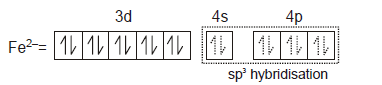*Multiple options can be correct
QUESTION: 3

### Which of the following complexes is (are) paramagnetic ?

Solution:

[Mn(CN)6]4- and [Cr(NH3)6]3+ contains 1 and 3 unpaired electrons respectively.
[Mn(CN)6]4- undergoes d²sp³ hybridization and is low spin complexes. It contains 1 unpaired electron each. Hence, it is paramagnetic in nature.
[Cr(NH3)6]3+ undergoes d²sp³ hybridization and is a low spin complex. It contains 3 unpaired electrons. Hence, it is paramagnetic in nature.

*Multiple options can be correct
QUESTION: 4

Which of the following statement(s) is(are) correct for coordination compound K2[Cr(NO+)(NH3)(CN)4]?

Solution:

According to the rules of A is correct and since nitrosonium ion is a SFL so pairing will happen.

*Multiple options can be correct
QUESTION: 5

Which of the following compounds/complex ions would not show geometrical isomerism?

Solution: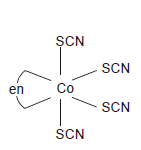exist in only one form.

*Multiple options can be correct
QUESTION: 6

In which of the following pair(s) both the complex show optical isomerism ?

Solution: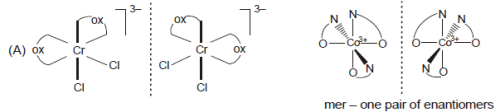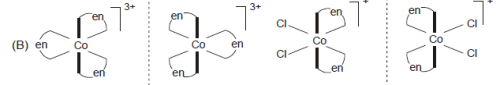(C) [Pt CI(dien)]CI , [Ni CI2 (PPh3)2] complex is square planar whereas [Ni CI2 (PPh3)2] is
tetrahedral.
(D) [Co (NO3)3 (NH3)3] has plane of symmetry but cis- [Pt (en)2 CI2] CI2 shows optical isomerism

QUESTION: 7

Double salts are addition compounds which lose their identity in aqueous solution whereas complexes which are also compounds do not lose their identity in aqueous solution. The coordination compounds show isomerism and find applications in photography, qualitative analysis, metallurgy, water purification and in the treatment of various diseases.

Q.

Which of the following statement is incorrect?

Solution:

It is homogenious catalyst for hydrogenation of alkenes.

QUESTION: 8

Double salts are addition compounds which lose their identity in aqueous solution whereas complexes which are also compounds do not lose their identity in aqueous solution. The coordination compounds show isomerism and find applications in photography, qualitative analysis, metallurgy, water purification and in the treatment of various diseases.

Q.

Which of the following statement is true for complex, [Co(NH3)4Br2]NO2 ?

Solution: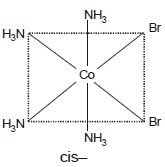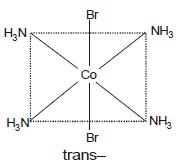It shows geometrical isomerism but not optical isomerism due to the presence of at least
one plane of symmetry.
[Co(NH3)4Br2]NO2 and [Co(NH3)4BrNO2]Br are ionisation isomers and later complexreacts
withAgNO3 solution to give pale yellow ppt. [Co(NH3)4BrNO2]Br and [Co(NH3)4BrONO]Br

QUESTION: 9

Double salts are addition compounds which lose their identity in aqueous solution whereas complexes which are also compounds do not lose their identity in aqueous solution. The coordination compounds show isomerism and find applications in photography, qualitative analysis, metallurgy, water purification and in the treatment of various diseases.

Q.

Choose the correct option for the complex [PtCl2(en)2]2+ .

Solution: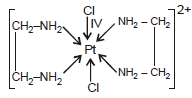Trans-form is optically inctive as it is achiral.

QUESTION: 10

In coordination chemistry there are a variety of methods applied to find out the structures of complexes. One method involves treating the complex with known reagents and from the nature of reaction, the formula of the complex can be predicted. An isomer of the complex Co(en)2(H2O)Cl2B4, on reaction with concentrated H2SO4 (dehydrating agent) it suffers loss in weight and on reaction with AgNO3 solution it gives a white precipitate which is soluble in NH3(aq).

Q.

The correct formula of the complex is :

Solution:

As it suffer lose in weight with H2SO4, ionization sphere spther3e contains H2O molecle.
As complex also give white ppt. with AgNO3, the ionisation sphere should contain CI– ion.
So formula may be [CoBrCI(en)2]CI.H2O.

QUESTION: 11

In coordination chemistry there are a variety of methods applied to find out the structures of complexes. One method involves treating the complex with known reagents and from the nature of reaction, the formula of the complex can be predicted. An isomer of the complex Co(en)2(H2O)Cl2B4, on reaction with concentrated H2SO4 (dehydrating agent) it suffers loss in weight and on reaction with AgNO3 solution it gives a white precipitate which is soluble in NH3(aq).

Q. If all the ligands in the coordination spheres of the above complex be replaxed by SCN -, then the paramagnetic momentof the complex ion (due to spin only) will be:

Solution:

SCN is weak field ligand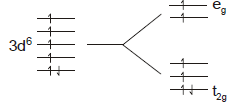no. of unpaired electrons = 4 ; 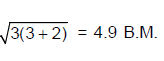QUESTION: 12

Co2+ (aq) + SCN- (aq.) →Complexe (X).Ni2+ (aq) + Dimetyhlglyoxime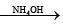Complex (Y).The coordination number of cobalt and nickel in complexes X and Y are four.

Q.

The IUPAC names of the complexes  (X) and (Y) are respectively

Solution:

(X) = [Co(SCN4)]2– ; (Y) = [Co(d,g)2].

QUESTION: 13

Co2+ (aq) + SCN- (aq.) →Complexe (X).
Ni2+ (aq) + DimetyhlglyoximeComplex (Y).
The coordination number of cobalt and nickel in complexes X and Y are four.

Q.

The geometry of complexes (X) and (Y) are respectively:

Solution:

X = [Co(SCcN)4]2– , cobalt is in +2 oxidation state and SCN is weak field ligand. So,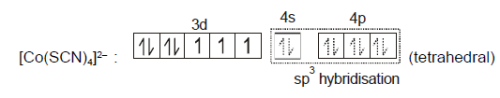[Ni(dmg)2] : Nickel is in +2 oxidation state and dmg is chelation ligand. So,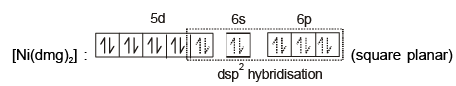QUESTION: 14

Co2+ (aq) + SCN- (aq.) →Complexe (X).
Ni2+ (aq) + Dimetyhlglyoxime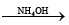Complex (Y).
The coordination number of cobalt and nickel in complexes X and Y are four.

Q.

Select the correct statement the complexes (X) and (Y)

Solution:

X = [Co(SCN)4]2– , cobalt is in +2 oxidation state and SCN is weak field ligand. So,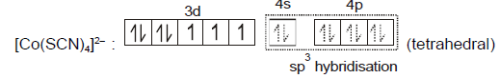[Ni(dmg)2] : Nckel is in +2 oxidationstate and dmg is chelating ligand. So,[Ni(dmg)2] shows intra moelcular H-bondign as shown below.
Reactions involved :

Co2+ (aq) + 4SCN (aq) →[Co(SCN)4]2– (aq.) blue colour complex.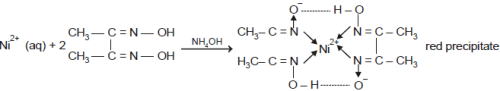QUESTION: 15

Crystal field theory views the bonding in complexes as arising from electrostatic interaction and considers the effect of the ligand charges on the energies of the metal ion d-orbitals.In this theory, a ligand lone pair is modelled as a point negative charge that repels electrons in the d-orbitals of the central metal ion. The theory concentrated on the resulting splitting of the d-orbitals in two groups with different energies and used that splitting to rationalize and correlate the optical spectra, thermodynamic stability, and magnetic properties of complexes. This energy splitting between the two sets of dorbitals is called the crystal field splitting D.
In general, the crystal field splitting energy D corresponds to wavelength of light in visible region of the spectrum, and colours of the complexes can therefore be attributed to electronic transition between the lower-and higher energy sets of d-orbitals.
In general, the colour that the we see is complementry to the colour absorbed.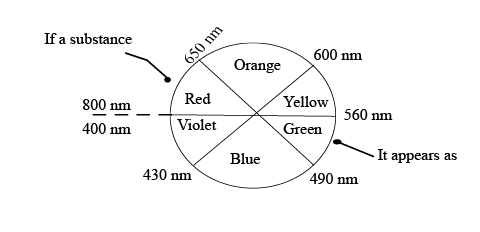Different metal ion have different values of D, which explains why their complexes with the same ligand have different colour.
Similarly, the crystal field splitting also depends on the nature of ligands and as the ligand for the same metal varies from H2O to NH3 to ethylenediamine, D for complexes increases. Accordingly, the electronic transition shifts to higher energy (shorter wavelength) as the ligand varies from H2O to NH3 to en, thus accounting for the variation in colour.
Crystal field theory accounts for the magnetic properties of complexes in terms of the relative values of   and the spin pairing energy P. Small    values favour high spin complexes, and large Dvalues favour low spin complexes.

The [Ti(NCS)6]3- ion exhibits a single absorption band at 544 nm. W hat will be the crystal field splitting energy (KJ mol-1) of the complex ? (h = 6.626 x 10-34 J.s ; C = 3.0 x 108 m/s; NA = 6.02 x 1023 ions/mole.

Solution: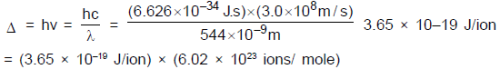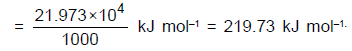QUESTION: 16

Crystal field theory views the bonding in complexes as arising from electrostatic interaction and considers the effect of the ligand charges on the energies of the metal ion d-orbitals.In this theory, a ligand lone pair is modelled as a point negative charge that repels electrons in the d-orbitals of the central metal ion. The theory concentrated on the resulting splitting of the d-orbitals in two groups with different energies and used that splitting to rationalize and correlate the optical spectra, thermodynamic stability, and magnetic properties of complexes. This energy splitting between the two sets of dorbitals is called the crystal field splitting D.
In general, the crystal field splitting energy D corresponds to wavelength of light in visible region of the spectrum, and colours of the complexes can therefore be attributed to electronic transition between the lower-and higher energy sets of d-orbitals.
In general, the colour that the we see is complementry to the colour absorbed.Different metal ion have different values of D, which explains why their complexes with the same ligand have different colour.
Similarly, the crystal field splitting also depends on the nature of ligands and as the ligand for the same metal varies from H2O to NH3 to ethylenediamine, D for complexes increases. Accordingly, the electronic transition shifts to higher energy (shorter wavelength) as the ligand varies from H2O to NH3 to en, thus accounting for the variation in colour.
Crystal field theory accounts for the magnetic properties of complexes in terms of the relative values of   and the spin pairing energy P. Small    values favour high spin complexes, and large Dvalues favour low spin complexes.

Which of the following statements is incorrect?

Solution:

As it absorbs blue colour light (λ = 455 nm), the colour of the complex must be orange.

QUESTION: 17

Crystal field theory views the bonding in complexes as arising from electrostatic interaction and considers the effect of the ligand charges on the energies of the metal ion d-orbitals.In this theory, a ligand lone pair is modelled as a point negative charge that repels electrons in the d-orbitals of the central metal ion. The theory concentrated on the resulting splitting of the d-orbitals in two groups with different energies and used that splitting to rationalize and correlate the optical spectra, thermodynamic stability, and magnetic properties of complexes. This energy splitting between the two sets of dorbitals is called the crystal field splitting D.
In general, the crystal field splitting energy D corresponds to wavelength of light in visible region of the spectrum, and colours of the complexes can therefore be attributed to electronic transition between the lower-and higher energy sets of d-orbitals.
In general, the colour that the we see is complementry to the colour absorbed.Different metal ion have different values of D, which explains why their complexes with the same ligand have different colour.
Similarly, the crystal field splitting also depends on the nature of ligands and as the ligand for the same metal varies from H2O to NH3 to ethylenediamine, D for complexes increases. Accordingly, the electronic transition shifts to higher energy (shorter wavelength) as the ligand varies from H2O to NH3 to en, thus accounting for the variation in colour.
Crystal field theory accounts for the magnetic properties of complexes in terms of the relative values of   and the spin pairing energy P. Small    values favour high spin complexes, and large Dvalues favour low spin complexes.

Which of the following complexes are diamagnetic ? [Pt(NH3)4]2+ [Co(SCN)4]2- [Cu(en)2]2+ [HgI4]2-square planar tetrahedral square planar tetrahedral       (i)       (ii)        (iii)     (iv)

Solution:

(i) 78Pt(II)has 5d8 configuration, all electrons are paired; so diamagnetic.
(ii) 27Co2+ has 3d7 configuration ; SCN is weak field ligand. So the complex is
paramagnentic with three unpaired electrons.
(iii) 29Cu2+ has 3d9 configuration; complex is paramagnetic with one unpaired electron.
(iv) 80Hg2+ has 5d10 condigukration ; all electrons are paired so diamagnentic.

QUESTION: 18

In metal caronyls, there is synergic bonding interaction between metal and carbon monoxide. This leads to increase in strength of metal ligand bond and decrease in bond order of CO in carbonyl complex as compared to bond order in carbon monoxide.
Simple carbonyls are invariably spin-paired complexes except for vanadium metal.

Q.

The increase in bond length in CO as compared to carbon monoxide is due to :

Solution:

Donation of electrons from a filled d-orbital of metal into the vacant antibonding π *
bonding orbital of CO decreases the bonbd order three to two and half or slightly more
thus increasing the bond length between C–O.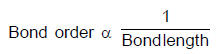QUESTION: 19

In metal caronyls, there is synergic bonding interaction between metal and carbon monoxide. This leads to increase in strength of metal ligand bond and decrease in bond order of CO in carbonyl complex as compared to bond order in carbon monoxide.
Simple carbonyls are invariably spin-paired complexes except for vanadium metal.

Q. Which amongst the following metal carbonyls are inner orbital complexes with diamagnetic property?

Solution:

Ni (CO)4 sp3 diamagnetic
Fe (CO)5 dsp3 diamagnetic
V (CO)6 dsp3 paramagnetic
Cr(CcO)6 dsp3 diamagnetic

QUESTION: 20

In metal caronyls, there is synergic bonding interaction between metal and carbon monoxide. This leads to increase in strength of metal ligand bond and decrease in bond order of CO in carbonyl complex as compared to bond order in carbon monoxide.
Simple carbonyls are invariably spin-paired complexes except for vanadium metal.

Q.

Which one of the following metal carbonyls involves the d2sp3?hybridisation for the formation of metal-carbon?? bonds and is paramagnetic.

Solution: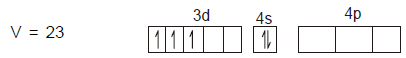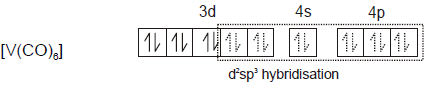QUESTION: 21

Match the ligands listed in column –I with the characteristic(s) type of ligands listed in column-II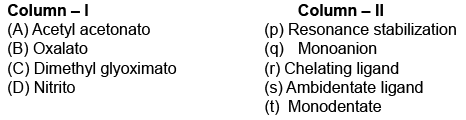Solution:

(A) Acetyl acetonato (CH3COCHCOCH3)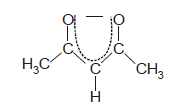(B) Oxalato (C2O42–)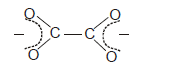(C) Dimethylglyoximato (HONCC(CH3)C(CH3)NO)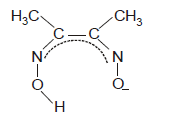(D) NItrito (NO2)
It may Ink to central metal ion either through nitrigen or oxygen; so it is ambidentate ligand.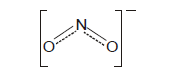QUESTION: 22

Match the complexes / complex ions given in column – I with the characteristic(s) given in column-II.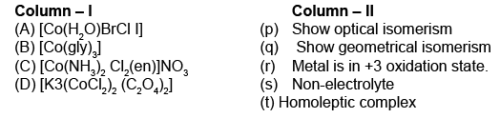Solution:

(A) It has tetrahedral geometry; so it does not show gemometrical isomerism. but it has chiral
atom and therefore, have a pair of enantiomers. Oxidation state of cobalt in the complex is
+3 and the compound is beteroleptic (having differe3nt types of ligands) and neutral.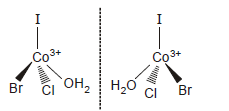(B) Oxidation staat3e of cobalt in the complex is +3 and the compound is homoleptic (having
same type of ligands) and neutral.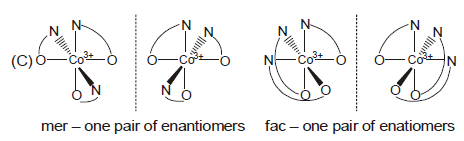(C) Oxidation state of cobalt in the complex is +3 and the compound is heteroleptic (having different
types of ligands).
[Co (NH3)2CI2(en)]NO3 (aq) ⇔[Co (NH3)2CI2(en)]+ (aq) + NO3(ap)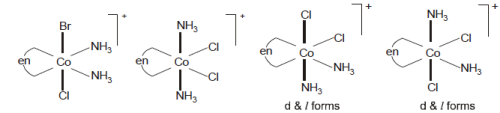(D) Oxidation state of cobalt in the complex is +3 and the compound is beteroleptic (having
different types of ligands).
K<[CoCI2(C2O4)2] (aq) ⇔3K+ (aq)+ [CoCI2 (C2O4)21]3– (aq)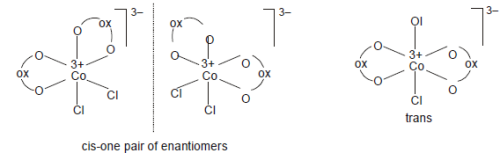QUESTION: 23

The volume (in mL) of 0.1 M AgNO3 required for complex precipitation of chloride ions present in 30 mL of 0.01 M solution of [Cr(H2O)5Cl]Cl2, as silver chloride is close to

Solution:
QUESTION: 24

Match the complexes listed in column – I with the characteristic(s) type of hybridisation listed in column-II.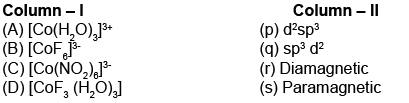Solution:

A. Sp3d2 and will be paramagnetic since water is WFL
B. 3d6 configuration, d2sp3 hybridization. This arrangement has a very large CFSE. Most of the ligands are strong enough to cause spin pairing. Such complexes are diamagnetic. The one exception is [CoF6]3– which is a high spin complex and is paramagnetic
C. NO2 strong field ligand, so compels for pairing of electron and thus it is diamagnetic with d2sp3 hybridization
D. Paramagnetic with sp3d2 hybridization

QUESTION: 25

Match the complexes listed in column – I with type hybridisation listed in column-II.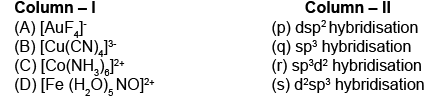Solution:
1. [AuF4]−, Au is in +3 oxidation state with 5d8 configuration, so complex is diamagnetic with dsp2 hybridization.

2. [Cu(CN)4]3−, Cu is in +1 oxidation state with 3d10 configuration, so complex is diamagnetic with sp3 hybridization.

3. [Co(NH3)6]3+, Co3+ has d5 configuration which has higher CFSE, so hybridization of d2sp3 and complex is diamagnetic.

4. [Fe(H2O)5NO]2+, Fe is in +1 oxidation state having 3d7 configuration, so hybridization of complex is sp3d2 and paramagnetic with three unpaired electrons.

Hence A is correct.